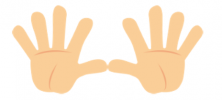Smartick is an advanced online program that teaches kids math and coding in only 15 min. a day

Mar11

# Tens and the Decimal Number System

Let’s begin this post by defining tens. A ten is a group of ten units. It is the most natural number in our body through the fingers on our hands. If we count them one by one, we can see that we have ten fingers (units), which is the same as saying we have a ten of fingers.We can express it in the following way:

10 units = 1 ten

10U = 1T

In our day to day life we use many numbers and therefore, we need a way to express them. They allow us to carry out calculations or represent large quantities, among other things.

To express these numbers we can find different number systems which use digits, elements, symbols or figures. Today, we will talk about one specific system related to tens: the decimal number system.

### What is the decimal number system?

This is a system that uses the number 10 as a base (that’s where we get the name ‘decimal’). It has a series of symbols that are used to represent all quantities. Surely you know these symbols because they the numbers 0, 1, 2, 3, 4, 5, 6, 7, 8, 9.

It is a positional system because each of the numbers is associated with a specific value depending on the space it occupies.

Therefore:

1 UNIT (1U)

1 TEN (1T) = 10U

1 HUNDRED(1H) = 10T = 100U

1 UNIT OF ONE THOUSAND (1UT) = 10H = 100T = 1.000U

1 TEN OF ONE THOUSAND (1TT) = 10UT = 100H = 1.000T = 10.000U

1 ONE HUNDRED OF ONE THOUSAND (1HT) = 10TT = 100UT = 1.000H = 10.000T = 100.000U

### A practical example using the decimal number system

We are going to complete an example using the number 629. In the decimal system, it is constructed in the following way, with respect to the corresponding positions:

(6 x 100) + (2 x 10) + (9 x 1)

600 + 20 + 9

629

As we can see, from right to left, the first place corresponds to the unit (9), the second place corresponds to the tens (20), and the third place corresponds to the hundreds (600).

If you need to, you can review the concept of units, tens, and hundreds, and then put it into practice everything you learned with Smartick!